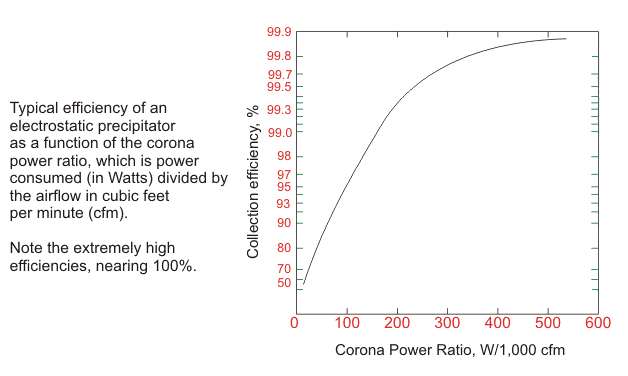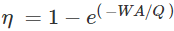# Electrostatic Precipitator Efficiency

Electrostatic precipitators have become the norm now in industries. Due to strict regulation and ever-increasing air pollution, installing one in a thermal power plant or any other power plants where flue gases are released, have become a necessity. But whether electrostatic precipitators perform the function expected from them can be determined by measuring the efficiency of the device. Different industries have different efficiency requirement. We are going to figure out a way to find the efficiency of the electrostatic precipitator.

The following factors affect the efficiency of an electrostatic precipitator.

## Corona Power Ratio

Before we go into the efficiency of an electrostatic precipitator, let us first understand what corona power ratio is (not to be confused with corona discharge). Corona power ratio is the ratio of power consumed in watts to the airflow in cubic feet per minute. This tells us about the energy consumed in filtering one cubic foot of air per minute. The corona power ratio affects the efficiency of an electrostatic precipitator. Higher the corona power ratio, higher is the efficiency of the electrostatic precipitator. The below image shows the variation of the efficiency of electrostatic precipitator with corona power ratio.## The Resistivity of Dust Collected

The efficiency of an electrostatic precipitator is dependent on its ability to collect the dust from the flue gases. The efficiency of collection of dust depends on its electrical resistivity. Particles with their resistivity in the normal zone are collected very easily by electrostatic precipitators. Dust collecting efficiency gets reduced in the case of particles which fall in the low resistivity zone, and they shed their charge as they arrive at the collecting plates, and they again reenter in the dust collection area. This phenomenon is called re-trainment. Even for particles in the high resistivity area, increasing electrical resistivity reduces the efficiency. Therefore the electrical resistivity of the particle dramatically affects the efficiency of the electrostatic precipitator.

## The Particle Size

The efficiency of the electrostatic precipitator is dependent on the particle size of the aerosol (dust, mist) to be collected. The collection efficiency if high for more massive particles and low for small particles.

The formula to calculate the efficiency
The Deutsch-Anderson equation gives the efficiency of an electrostatic precipitator, and the equation is as follows:η = fractional collection efficiency
W = terminal drift velocity in m/s
A = total collection area in m2
Q = volumetric air flow rate in m3/s
We are not going into the derivation of the formula but will only try to understand the meaning of it.
Terminal drift velocity is the velocity an object attains when it falls through the air (or any other medium). Total collection area here denotes the entire area of the collecting plates. Volumetric air flow rate is the volume of gas which passes per unit time. Using the above equation, we can find out the fractional collection efficiency of an electrostatic precipitator.

Want To Learn Faster? 🎓
Get electrical articles delivered to your inbox every week.
No credit card required—it’s 100% free.